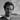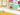Povel Croona, Learning Designer

9 September 2020

# JavaScript Countdown Timer in 14 Lines

Countdown timers are useful in a lot of scenarios. Your marketing colleagues may use them to stimulate the purchase; one-time password/two-factor authentication solutions benefit from extra transparency; clock is better than a fixed time for international milestones. Just like they eliminate the confusion, we’re about to clue you in on how to make a JavaScript countdown timer yourself.## JavaScript Countdown Timer Logic

In the nutshell, here’s what you would like to with a JavaScript countdown timer:

1. Define the end date
2. Pull the current date
3. Calculate the distance between the two dates
4. Set the displayed distance to update every second
5. Code in a message that displays at the end of the count down
6. Output the countdown

This basic logic makes the countdown timer relevant for users all over the world and keeps it ticking even when the user has not interacted with the page for a while. Like I mentioned earlier, this is a very popular way to trick people into impulse purchases. Even if you take a few minutes to discuss the potential purchase with someone at home, you come back to the same screen that now has even less time left on the clock.

## JavaScript Countdown Timer: Vanilla JavaScript

Here’s how you would make a JavaScript countdown timer to a date if you’re stuck with the vanilla JavaScript.

``````let countDownDate = new Date("Jan 01, 2021 00:00:00").getTime(); // The deadline
let x = setInterval(() => {
let now = new Date().getTime();
let distance = countDownDate - now;

let days = Math.floor(distance / (1000 * 60 * 60 * 24));
let hours = Math.floor((distance % (1000 * 60 * 60 * 24)) / (1000 * 60 * 60));
let minutes = Math.floor((distance % (1000 * 60 * 60)) / (1000 * 60));
let seconds = Math.floor((distance % (1000 * 60)) / 1000);
console.log(days + "d " + hours + "h " + minutes + "m " + seconds + "s"); // Countdown output

if (distance < 0) {
clearInterval(x);
console.log("Too late!") // Text at the end of the countdown
}
}, 1000);``````

## JavaScript Countdown Timer: jQuery

This library has a very neat plugin jQuery.countdown, so making a countdown timer here will be a piece of cake. Here are a couple of examples from the documentation.

### Days+HH:MM:SS to a Date

#### HTML

``<span id="clock"></span>``

#### JavaScript

``````\$('#clock').countdown('2021/01/01', function(event) {
\$(this).html(event.strftime('%D days %H:%M:%S'));
});``````

#### HTML

``````<div class="countdown">
3 hours to secure your pre-order! <!--Message alongside the countdown-->
<span id="clock"></span>
</div>``````
``````\$('#clock').countdown('2020/01/01 00:00:00')
.on('update.countdown', function(event) {
var format = '%H:%M:%S';
if(event.offset.totalDays > 0) {
format = '%-d day%!d ' + format;
}
if(event.offset.weeks > 0) {
format = '%-w week%!w ' + format;
}
\$(this).html(event.strftime(format));
})
.on('finish.countdown', function(event) {
\$(this).html('Pre-orders are gone!') // Expiration message

});``````

## JavaScript Countdown Timer: React

Here, we will create a JavaScript countdown timer with the JSX syntax extension.

``````import React, { Component } from "react";

class Timer extends Component {
constructor(props) {
super(props);
this.countDownId = null;
this.state = {
days: 0,
hours: 0,
minutes: 0,
seconds: 0,
expired: false
};
}

componentDidMount() {
this.countDownId = setInterval(this.timerInit, 1000);
}

componentWillUnmount() {
if (this.countDownId) {
clearInterval(this.countDownId);
}
}

timerInit = () => {
const { startDate } = this.props;
console.log(startDate);
const now = new Date().getTime();
if (!startDate) {
this.setState({ expired: true });
return;
}
const countDownStartDate = new Date(startDate).getTime();
const distance = countDownStartDate - now;
const days = Math.floor(distance / (1000 * 60 * 60 * 24));
const hours = Math.floor(
(distance % (1000 * 60 * 60 * 24)) / (1000 * 60 * 60)
);
const minutes = Math.floor((distance % (1000 * 60 * 60)) / (1000 * 60));
const seconds = Math.floor((distance % (1000 * 60)) / 1000);

if (distance < 0) {
clearInterval(this.countDownId);
this.setState({
days: 0,
hours: 0,
minutes: 0,
seconds: 0,
expired: true
});
return;
}
this.setState({ days, hours, minutes, seconds, expired: false });
};

render() {
const { days, hours, minutes, seconds, expired } = this.state;
if (expired) {
return <div className="expired">Expired :(</div>;
}
return (
<div className="timer">
<div>
{days}
<span>d</span>
</div>
<div>
{hours}
<span>h</span>
</div>
<div>
{minutes}
<span>m</span>
</div>
<div>
{seconds}
<span>s</span>
</div>
</div>
);
}
}
export default Timer;``````

Let's import our timer and utilize it for a countdown that ends within an hour of the user loading the page/screen.

``````import React from "react";
import ReactDOM from "react-dom";

import "./styles.css";

import Timer from "./Timer";

function App() {
const startDate = new Date().getTime() + 3600000; // 1 hour in milliseconds
return (
<div className="App">
<h1>Timer:</h1>
<Timer startDate={startDate} />
</div>
);
}

const rootElement = document.getElementById("root");
ReactDOM.render(<App />, rootElement);``````

We don't need a stressful timer to announce that our autumn Frontend Development group starts studying on November 30. We teach HTML, CSS, and JavaScript essentials to get you started as a Junior Developer in 4 months. Find out more and grab your spot here.